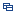| Recent Visit History | Join Audited Suppliers | Help | Region Guide
» » Jilin Product List
801.

Bi-Convex Cylindrical Lenses with Bk7 Material[Jan 20, 2022]

Plano-convex is close to best form for a focusing lens. Focusing or optical imaging Focal Length: F = R/(n-1) N: Refractive index R: Radius curvature Plano-convex lenses are useful for a wide range of ...

802.

Bi-Convex Cylindrical Lenses for Laser Use00[Jan 20, 2022]

Plano-convex is close to best form for a focusing lens. Focusing or optical imaging Focal Length: F = R/(n-1) N: Refractive index R: Radius curvature Plano-convex lenses are useful for a wide range of ...

803.

Bi-Convex Cylindrical Lenses Made in China[Jan 20, 2022]

Plano-convex is close to best form for a focusing lens. Focusing or optical imaging Focal Length: F = R/(n-1) N: Refractive index R: Radius curvature Plano-convex lenses are useful for a wide range of ...

804.

Bi-Convex Cylindrical Lenses with Optical Glass Material[Jan 20, 2022]

Plano-convex is close to best form for a focusing lens. Focusing or optical imaging Focal Length: F = R/(n-1) N: Refractive index R: Radius curvature Plano-convex lenses are useful for a wide range of ...

805.

Bi-Convex Cylindrical Lenses for Wholesale[Jan 20, 2022]

Plano-convex is close to best form for a focusing lens. Focusing or optical imaging Focal Length: F = R/(n-1) N: Refractive index R: Radius curvature Plano-convex lenses are useful for a wide range of ...

806.

Bi-Convex Cylindrical Lenses with Zygo Report[Jan 20, 2022]

Plano-convex is close to best form for a focusing lens. Focusing or optical imaging Focal Length: F = R/(n-1) N: Refractive index R: Radius curvature Plano-convex lenses are useful for a wide range of ...

807.

Bi-Convex Cylindrical Lenses with Hr Coating[Jan 20, 2022]

Plano-convex is close to best form for a focusing lens. Focusing or optical imaging Focal Length: F = R/(n-1) N: Refractive index R: Radius curvature Plano-convex lenses are useful for a wide range of ...

808.

Bi-Convex Cylindrical Lenses with Jgs3coating[Jan 20, 2022]

Plano-convex is close to best form for a focusing lens. Focusing or optical imaging Focal Length: F = R/(n-1) N: Refractive index R: Radius curvature Plano-convex lenses are useful for a wide range of ...

809.

Bi-Convex Cylindrical Lenses with Jgs2 Material[Jan 20, 2022]

Plano-convex is close to best form for a focusing lens. Focusing or optical imaging Focal Length: F = R/(n-1) N: Refractive index R: Radius curvature Plano-convex lenses are useful for a wide range of ...

810.

Bi-Convex Cylindrical Lenses Wih Baf2 Material[Jan 20, 2022]

Plano-convex is close to best form for a focusing lens. Focusing or optical imaging Focal Length: F = R/(n-1) N: Refractive index R: Radius curvature Plano-convex lenses are useful for a wide range of ...

811.

Bi-Convex Cylindrical Lenses with Bbar Coating[Jan 20, 2022]

Plano-convex is close to best form for a focusing lens. Focusing or optical imaging Focal Length: F = R/(n-1) N: Refractive index R: Radius curvature Plano-convex lenses are useful for a wide range of ...

812.

Bi-Convex Cylindrical Lenses with Jgs2 Material[Jan 20, 2022]

Plano-convex is close to best form for a focusing lens. Focusing or optical imaging Focal Length: F = R/(n-1) N: Refractive index R: Radius curvature Plano-convex lenses are useful for a wide range of ...

813.

Bi-Convex Cylindrical Lenses with CaF2 Coating[Jan 20, 2022]

Plano-convex is close to best form for a focusing lens. Focusing or optical imaging Focal Length: F = R/(n-1) N: Refractive index R: Radius curvature Plano-convex lenses are useful for a wide range of ...

814.

Bi-Convex Cylindrical Lenses with Coating[Jan 20, 2022]

Plano-convex is close to best form for a focusing lens. Focusing or optical imaging Focal Length: F = R/(n-1) N: Refractive index R: Radius curvature Plano-convex lenses are useful for a wide range of ...

815.

Bi-Convex Cylindrical Lenses with Jgs1 Material[Jan 20, 2022]

Plano-convex is close to best form for a focusing lens. Focusing or optical imaging Focal Length: F = R/(n-1) N: Refractive index R: Radius curvature Plano-convex lenses are useful for a wide range of ...

816.

Bi-Convex Cylindrical Lenses for Hot Sale[Jan 20, 2022]

Plano-convex is close to best form for a focusing lens. Focusing or optical imaging Focal Length: F = R/(n-1) N: Refractive index R: Radius curvature Plano-convex lenses are useful for a wide range of ...

817.

Bi-Convex Cylindrical Lenses for Laser Use[Jan 20, 2022]

Plano-convex is close to best form for a focusing lens. Focusing or optical imaging Focal Length: F = R/(n-1) N: Refractive index R: Radius curvature Plano-convex lenses are useful for a wide range of ...

818.

Bi-Convex Cylindrical Lenses Made in China[Jan 20, 2022]

Plano-convex is close to best form for a focusing lens. Focusing or optical imaging Focal Length: F = R/(n-1) N: Refractive index R: Radius curvature Plano-convex lenses are useful for a wide range of ...

819.

Bi-Convex Cylindrical Lenses with Optical Glass Material[Jan 20, 2022]

Plano-convex is close to best form for a focusing lens. Focusing or optical imaging Focal Length: F = R/(n-1) N: Refractive index R: Radius curvature Plano-convex lenses are useful for a wide range of ...

820.

Bi-Convex Cylindrical Lenses with Zygo Report[Jan 20, 2022]

Plano-convex is close to best form for a focusing lens. Focusing or optical imaging Focal Length: F = R/(n-1) N: Refractive index R: Radius curvature Plano-convex lenses are useful for a wide range of ...

Hot Region Index: ABCDEFGHIJKLMNPQRSTUWXYZ